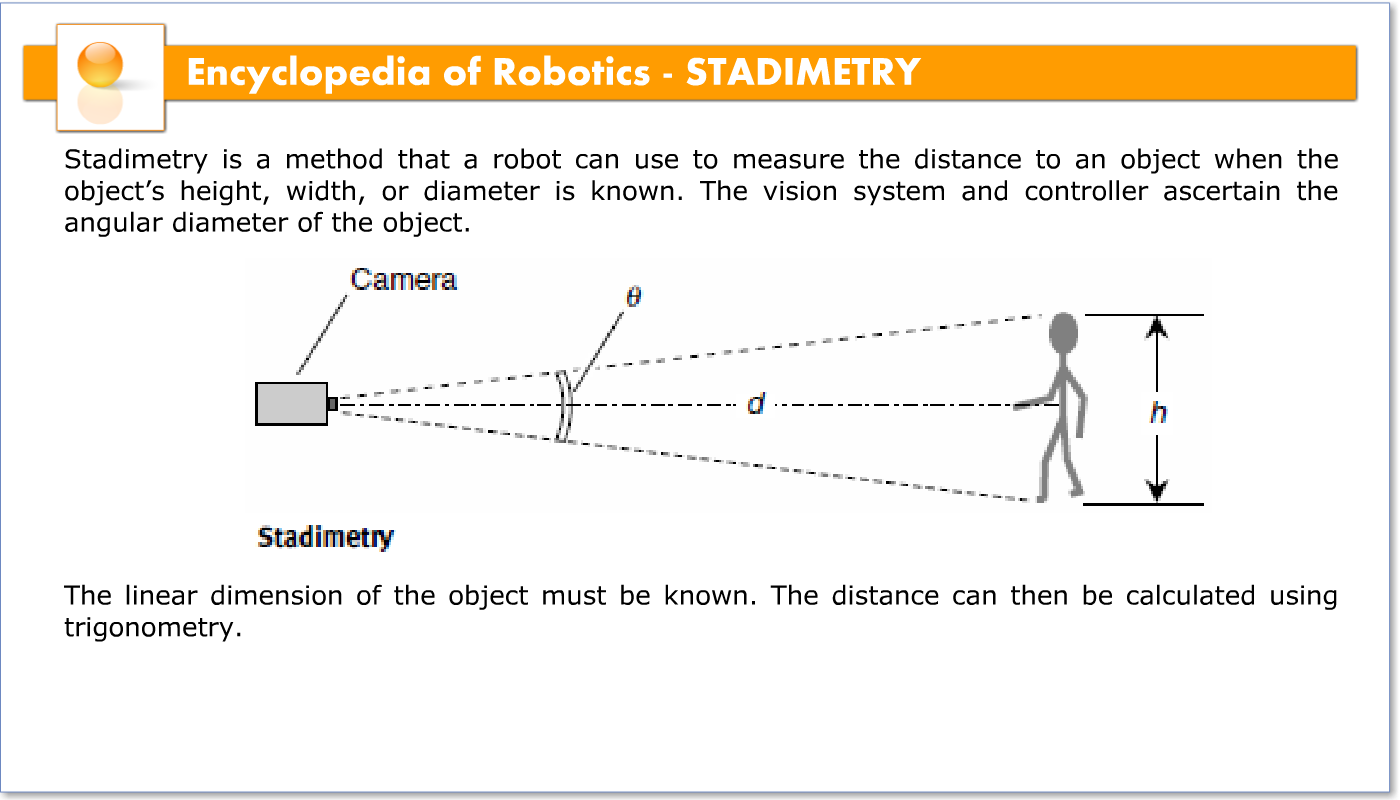Stadimetry is a method that a robot can use to measure the distance to an object when the object’s height, width, or diameter is known. The vision system and controller ascertain the angular diameter of the object. The linear dimension of the object must be known. The distance can then be calculated using trigonometry.The illustration shows an example of stadimetry as it might be used to measure the distance d, in meters, from a robot camera to a person. Suppose the person’s height h, in meters, is known. The vision system determines the angle that the person subtends in the field of view.From this information, the distance d is calculated according to the following formula:If the distance d is large compared with the height h, a simpler formula can be used:In order for stadimetry to be accurate, the linear dimension axis (in this case the axis that depicts the person’s height, h) must be perpendicular to a line between the vision system and the center of the object. Also, it is important that d and h be expressed in the same units.